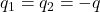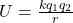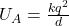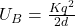## In case A two negative charges which are equal in magnitude are separated by a distance d. In case B the same charges are separated by a dis

Question

In case A two negative charges which are equal in magnitude are separated by a distance d. In case B the same charges are separated by a distance 2d. Which configuration has the highest potential energy

in progress 0
6 months 2021-07-29T09:26:48+00:00 1 Answers 6 views 0

Case A

Explanation:

We are given that

Case A:

LetDistance between two negative  charges,r=d

Case B:

Charges are same

Distance between two charges,r=2d

Potential energy=Using the formula

For case AFor case BPotential energy is inversely proportional to distance between two charges

The distance between two charges in case A is small therefore, it has the highest potential energy.